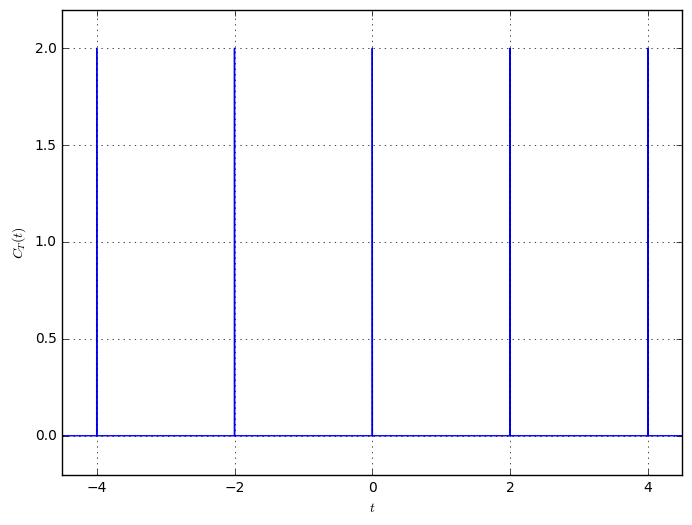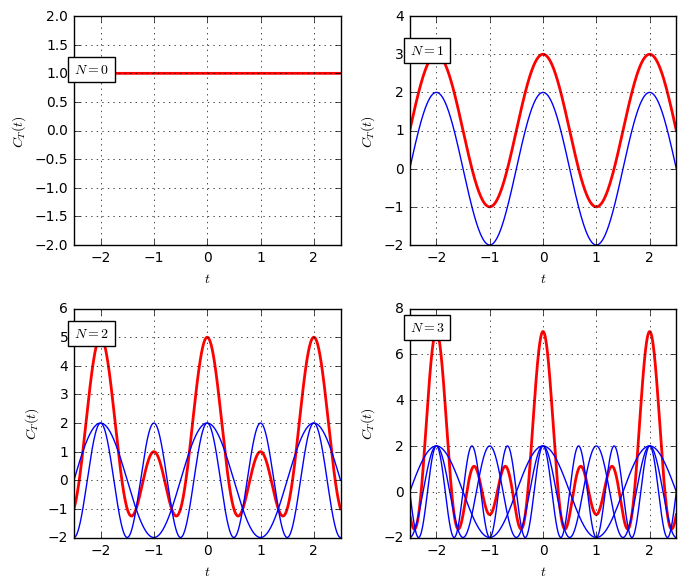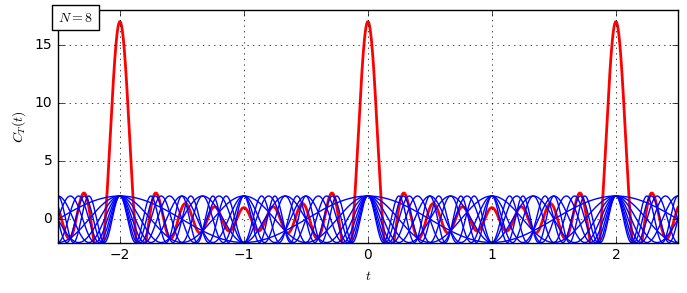The Dirac Comb function¶

The continuous-time comb function $C_T(t)$ is an important tool in signal processing and sampling theory and given by

$$C_T(t)=T\sum_{n=-\infty}^{\infty}\delta(t-nT).$$

Let us first draw this Dirac comb function. Due to the infinitely small width of the Dirac impulse, it is tough to numerically model the Dirac comb in continuous time. Let us therefore resort to discrete time for the visualization:

T = 2                      # time distance between pulses
Fs = 1000                  # sampling frequency, used for discretizing the system
t = np.arange(-6, 6, 1/Fs) # time range to consider
comb = np.zeros_like(t)
comb[::int(Fs*T)] = T     # Comb becomes T every T*Fs samples
plt.plot(t, comb)As shown, the Dirac comb is a periodic repetition of the Dirac impulse, where the distinct impulses are $T$ apart.

In this notebook we will look at different representations of the comb function and its Fourier transform. The relations in time- and frequency domain are nicely visualized by this diagram:The diagram shows, that the comb function can either be represented as a sum of Dirac impulses by $$C_T(t)=T\sum_{n=-\infty}^{\infty}\delta(t-nT)$$ or equivalently as a sum of complex exponentials that have frequencies $nt/T$: $$C_T(t)=\sum_{n=-\infty}^{\infty}\exp(j2\pi nt/T).$$

This representation might not be intuitive. Therefore, let us try to reproduce this equation numerically. First, let us see that we can reformulate this with the identity $$\cos(2\pi nt/T)=\frac{1}{2}\left(\exp(j2\pi nt/T)+\exp(-j2\pi nt/T)\right).$$ We summarize the exponential terms for positive and negative frequencies into a single cosine, and get $$C_T(t)=1+\sum_{n=1}^\infty2\cos(2\pi nt/T),$$ where the term $1$ corresponds to $1=\exp(j2\pi\cdot0t/T)=\exp(0)$.

Let us create a function that shows the sum of the first $N$ terms of the series and see how the resulting function looks like:

T = 2  # time-distance between diracs
Fs = 10000 # sampling frequency
def combApprox(N):
t = np.arange(-2.5, 2.5, 1/Fs)
sigSum = np.ones_like(t)
for n in range(1,N+1):
part = 2*np.cos(2*np.pi*n*t/T)
sigSum = sigSum + part
if n < 50:
plt.plot(t, part, 'b-')
plt.plot(t, sigSum, 'r-', lw=2, zorder=-1)

plt.figure(figsize=(7,6))
plt.subplot(221); combApprox(0)
plt.subplot(222); combApprox(1)
plt.subplot(223); combApprox(2)
plt.subplot(224); combApprox(3)

plt.figure(figsize=(8,3)); combApprox(8)As visible, the cosine-functions add up constructively at $t=nT$ to a high value. For $t\neq nT$, the different cosine waves have different signs and magnitudes and average out to become a small value close to zero. The more cosines are considered (i.e. higher $N$), the tighter and higher become the peaks of the sum.